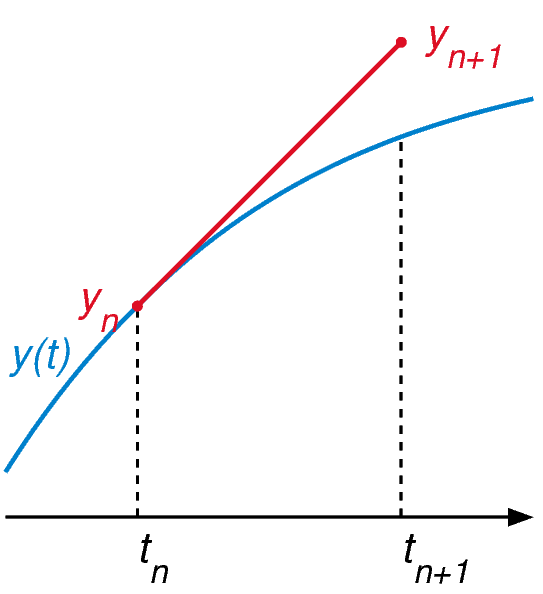# Our favourite (and not-so-favourite) Euler equations

A collection of our favourite and least favourite things named after Euler, from issue 07In previous issues of Chalkdust, we shared with you a selection of our favourite “things” in maths, such as our favourite functions, shapes and sets. On the other hand, there are also some things we find annoying and very much dislike, such as bad notation, certain numbers, etc. For this special occasion (commemorating Euler’s birthday Euler a few weeks ago), we decided to spread some of our favourite, and not-so-favourite, examples of things named after Euler throughout issue 07.

We would also like to hear yours! Send them to us at contact@chalkdustmagazine.com.

## Euler’s polyhedral formula (Emily Maw)

Euler’s polyhedral formula says that the number of vertices, $V$, edges, $E$, and faces, $F$, of a convex 3D polyhedron satisfy

$$V-E+F=2.$$

This is one of my favourite equations, because it’s arguably the first theorem in topology! Despite the name, Euler couldn’t actually prove it; the first of many proofs were found by Leibniz and Cauchy. It can be used to obtain strong constraints on the existence of shapes, for example in the proof that there are only five Platonic solids, or that all polyhedra built from pentagons and hexagons (such as footballs) must contain exactly 12 pentagons! The reason it holds for convex polyhedra is that they are all homeomorphic (topologically equivalent, by smoothing off the corners) to a sphere. In fact the formula can be generalised: for nonconvex polyhedra, which can be homeomorphic to surfaces with holes, the formula becomes $V-E+F=2-2g$, where $g$ is the genus (number of holes). This is because the left hand side is secretly calculating a topological invariant of the space, called the Euler characteristic, which is equal to $2-2g$ for surfaces.

## Euler’s identity (Matthew Scroggs)

My least favourite equation is

$$\mathrm{e}^{\,\mathrm{i} \,\pi}+1=0.$$

People rant about how it’s the most beautiful equation in maths, but it’s not. Overrated.

## Euler’s continued fraction formula (Nikoleta Kalaydzhieva)

Euler’s continued fraction formula connects some infinite series with an infinite continued fraction. For example, it gives the following result for $\mathrm{e}^z$:

$$\mathrm{e}^z={\cfrac{1}{1-\cfrac{z}{1+z-\cfrac{z}{2+z-\cfrac{2z}{3+z-\cfrac{3z}{4+z-\cdots}}}}}}$$

Isn’t it pretty?

## Euler’s method (Sean Jamshidi)

$$f\hspace{2pt}’\hspace{-2pt}(x) = \frac{f\hspace{1pt}(x+h) – f\hspace{1pt}(x)}{h}.$$

Euler’s method is a way of approximately solving differential equations using a computer, which is one of the most common tasks in applied mathematics. The method was invented hundreds of years before the computer, can be hopelessly inaccurate and is so simple that it has no right to be of any use at all. And yet… we still teach it, it can be coded up in 2 minutes and, in some cases, provides perfectly useable results. Sometimes the oldies are the best ones.As accurate as a sledgehammer, and about as good at maths. What am I? An engineer? Wikimedia Commons, CC BY 4.0

## The Euler-Lagrange equation (Tom Rivlin)

My favourite Euler equation is the Euler-Lagrange equation:

$$\frac{\partial L}{\partial x_i}- \frac{\mathrm{d}}{\mathrm{d}t} \Big( \frac{\partial L}{\partial \dot{x}_i} \Big) = 0$$

It’s the key equation of the calculus of variations, which is used extensively in many branches of physics. One uses the Euler-Lagrange equation to derive the Lagrangian of a physical system, from which all of its physical properties can be determined. A famous example is the Lagrangian of the standard model of particle physics, which is a one-equation summary of all of our current knowledge about the fundamental building blocks of reality:

$$\mathcal{L} = -\frac{1}{4} F_{\mu \, v} F^{\mu \, v} + \mathrm{i} \overline{\Psi} \mathrm{D} \Psi + h.c. +\Psi_i y_{ij}\Psi_j \Phi +h.c.+|D_{\mu}\Phi|^2 – V(\Phi )$$

## Incompressible Euler equations for inviscid fluids (Hugo Castillo)

$$\boldsymbol{\nabla}\cdot\boldsymbol{u}=0 \qquad \rho\left(\frac{\partial \boldsymbol{u}}{\partial t}+\boldsymbol{u}\cdot\boldsymbol{\nabla}\boldsymbol{u}\right) = -\boldsymbol{\nabla} P.$$

These equations, first published by Euler, are a set of coupled quasilinear partial differential equations widely used in fluid mechanics. They allow you to calculate the velocity and pressure fields in a moving, inviscid (zero-viscosity) fluid. I dislike them for the simple fact that they are only valid for water and are completely useless for cases when the fluid viscosity is highly important (eg my PhD research and 95% of the fluids present in this world).Paint, one of the many examples for which the Euler equations for inviscid fluids are not valid. Adapted from Flickr, CC BY 2.0.

## Euler’s solution to the Basel problem (Belgin Seymenoğlu)

$$\sum_{n=1}^{\infty}\frac{1}{n^2} = \frac{\pi^2}{6}.$$

I remember solving the problem by myself using Fourier Series as an undergrad, while revising for an exam. You can imagine my disappointment to find that Euler beat me to it during the mid-18th century!